Alph - A Little Process Helper

### Function Solver Tutorial

While a sound knowledge of process fundamentals and clever use of equations can often provide explicit solutions to problems that might require iterative loops in a modular simulator, sometimes you have to resort to some sort of iteration to get the answer you need. This tutorial will introduce the use of the Function Solver Tool to address to such problems.

If you have not yet worked through the previous tutorials, you might want to begin with them, but a completed tutorial 6 case is available.

Alph tutorial pages:

In this tutorial we shall:

• Use the Function Solver Tool to adjust the low temperature separator temperature to achieve a desired sales gas dew point in the gas plant from the previous tutorials.
• Add a second function to the solver and have it solve both simultaneously

Note: the use of the function solver for stream recycle problems will be addressed in the following Recycle Tutorial.

### Solver Basics

The function solver tool essentially attempts to solve problems of the form:

f(x) = 0

You supply the function or functions as formulas that should equate to zero at the solution. The solver has output values of x, which you use in formulas, either directly in the solver formulas, or more typically in formulas defining fluid or other values that are in turn indirectly referenced by the solver formulas.

Miscellaneous solver information:

• The allowable error in function values is 0.0001, so your error formulas should be scaled appropriately.
• Initially or whenever the solver is reset, the output values are all equal to zero.
• If there is only a single equation, the output value at the solution must lie in the range of -10 to 10. This is not required for multiple functions, but you should still scale your problem so the solution values will be roughly in that range.
• A single equation problem will use a Brent solution algorithm, while multiple equations are handled with Broyden.

If any (all?) of this seems confusing, hopefully the following examples will make it much clearer.

### Solver Example

For the first task, load the saved gas plant case used in earlier tutorials. If you don't have the case saved, you can obtain the completed tutorial 6 here. The flowsheet this represents is:Back in tutorial 3 we manually adjusted the low temperature separator temperature to roughly meet a desired sales gas dew point temperature. We will now use the function solver to automate this.The first thing we shall do is create a variable to hold the desired dew point temperature.

Go to the information diagram and tap the Add Var button to create the variable. Name it "dewspec", with a description if you wish. Set its Type to T and its value to -10 C.

Return to the information diagram and tap the Add Tool button to create the solver tool. Select Function Solver from the list of tool types.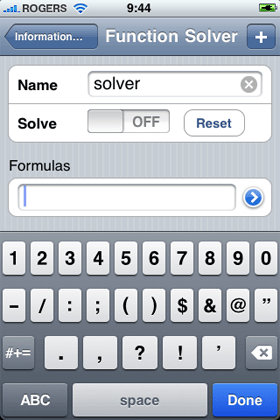In addition to the name field, the solver has a switch to turn it on and off, a button to reset all the outputs to zero and a single formula field (more formulas can be added by means of the plus button on the title bar).

For now, just name the tool solver and then tap the blue disclosure icon beside the formula field to open the formula editor.This is the standard formula editor page, but with the addition of a Select Type... button, which will allow you to select the property type for the formula, much as you would for an Alph variable.

Tap this button and select T from the resulting spinner.The formula we enter here should evaluate to zero when the solver is converged. In our case we simply want the temperature of the dewpt fluid to be equal to the specification in the dewspec variable, so enter

@dewpt - \$dewspec

In many cases you will probably want to scale this error, keeping in mind the 0.0001 tolerance for convergence, but that tolerance should work fine for a temperature difference.

Tap the Save button to return to the main function solver page.At the bottom of the solve page (tap the Done button on the keyboard if it is in the way), we can see that the error has been calculated and is already pretty small as might be expected as we have already manually picked an appropriate LTS temperature.

Before we enable the solver, we need to make use of its output to calculate the temperature of the lts fluid.

Go to the lts fluid page and open the formula editor for its temperature.Keeping in mind that the output value of the solver will initially be zero and will range from -10 to 10 and further that the lts temperature will probably be fairly close to the dew point temperature, the following formula should be appropriate:

\$dewspec + #solver.0

Function solver output values are referenced by just the formula number, starting with 0. You can also reference the calculated function values with f0, f1 etc.

Tap the Save button to return to the main lts page and then switch to the Bulk view.As the solver output is still 0, the temperature should be equal to the \$dewspec value, namely -10 C.

Return to the solver tool and tap the solve switch to attempt the solution.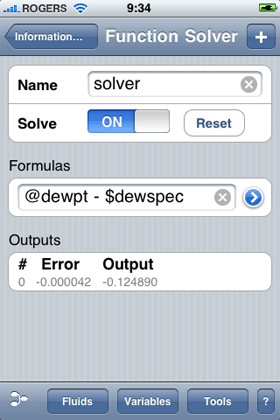This is an easy problem and the solver should quickly solve. Note the output value and resulting error are shown at the bottom. Switch to the dewpt fluid and confirm that its temperature is indeed -10 C (plus or minus 0.0001) and then check the lts fluid temperature, which should be about -10.125 C.

Try changing the dewspec variable to 0 C and then return to the dewpt fluid to confirm it has been recalculated appropriately.

Now let's try creating an error.

Change the dewspec temperature to 10 C.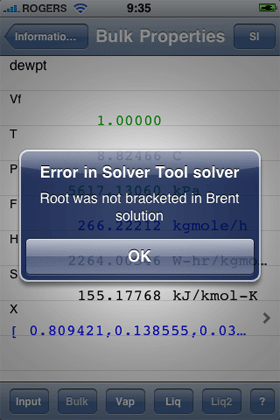You should get an error like the one shown to the right. When you clear it by tapping OK, you will see that the dew point is only 8.8 C, rather than the required 10 C. If you then check the lts fluid you will see that it is at 20 C, which corresponds to the specification plus the maximum output range for a single value solution. What is more the vapour fraction is 1, but the dew point is still less than 10 C. Clearly the spec we used cannot be met.

Return to the dewspec variable and set it back to -10 C and then return to the dewpt fluid. The dew point temperature will have changed, but not to the requested value. What is happening?

Whenever the solver encounters an error or is modified in any way, it disables itself to avoid repeated error messages etc. The dewpt value will have changed since the lts temperature will have changed to reflect the new dewspec value, but it will still be using the output of 10 from the solver, so its value will be 0.

Return to the solver and tap the Reset button. This will return the output value(s) to 0 and you should see the error value change from about 9.59 to 0.12 as the lts temperature changes accordingly.

Tap the Solve switch to again obtain a valid solution.Let's try adding another equation to solve, by making the rather dubious assumption that we have limited refrigeration compressor power and that we would actually reduce the lts pressure to compensate.

First create a new variable of type Q. Name it hpspec and give it value of 10000 W and then return to the solver and tap the + button on the tool bar to add another formula.

Open the new formula's editor page and choose Q as the type.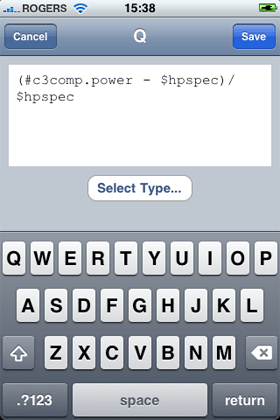This time the error will be the difference between the calculated power of c3comp and the spec. Enter the formula:

(#c3comp.power - \$hpspec) / \$hpspec

As the power value is a fairly large number, I have normalized it with the spec value.

Tap the Reset button to reinitialize the output values.Go the lts fluid and open the formula editor for pressure.Since the output value will start as zero, we will add one to it and then multiply that by the feed fluid pressure.

@feed * (1 + #solver.1)

This time we use #solver.1 to indicate the second output from the solver.

Go to the lts fluid bulk properties page.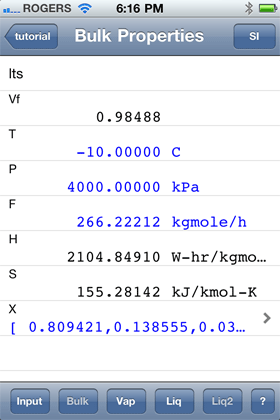Check that the temperature and pressure are being appropriately calculated for the initial output values.

Return to the solver page.This should be displaying both formulas along with the initial errors as shown to the right.

Tap the Solve switch.After some iteration, the solution should complete and return you to a page like this. Check the c3comp tool and the dewpt fluid to confirm that both specs have been met. If you check the lts fluid, you should see that a temperature of -10.74 C and a pressure of 3585 kPa was necessary to achieve the specifications.

The solver formulas used in this tutorial returned scalar values, but it is also possible to have formulas of type X which will add a number of equations equal to the number of compounds in the case to the solver. This, along with a simple quasi manual method of solving recycles, is explored in the recycle tutorial.